A 47.7-g golf ball is driven from the tee with an initial speed of 44.2 m/s and rises to a height of 25.9 m. (a) Neglect air resistance and

Question

A 47.7-g golf ball is driven from the tee with an initial speed of 44.2 m/s and rises to a height of 25.9 m. (a) Neglect air resistance and determine the kinetic energy of the ball at its highest point. (b) What is its speed when it is 7.73 m below its highest point

in progress 0
5 months 2021-08-06T10:22:36+00:00 1 Answers 650 views 0

(a) 34 J

(b) 40 m/s.

Explanation:

(a)As no air resistance, so total mechanical energy of golf ball remains constant.

i.e,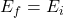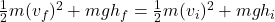Here m is the mass of the golf ball and g is the acceleration due to gravity.

Given m = 47.0 g= 0.047 kg ,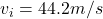,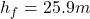and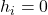(because ball start from zero.)

Substitute the given values, we get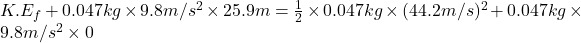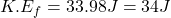Thus, the kinetic energy of the ball at its highest point is 34 J.

(b) when the ball is 7.73 m below its highest point then it final height,

25.9 m – 7.73 m =18.17 m.

Now from above,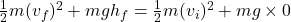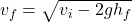Substitute the values, we get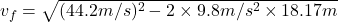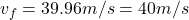Thus. final speed of ball when it is 7.73 m below its highest point is 40 m/s.